# Image Processing-Image Restoration

Posted by GwanSiu on December 27, 2017

In this article, image restoration and its techniques are introduced: (1). the basic concept of image restoration and its difference from image enhancement. (2).The inverse filter (3).The wiener filter (4). MAP formulation

Image restoration is to reconstruct the latent high quality image from its degraded observation. The acquired image is degraded observation of the unknown latent image, while degradation comes from various factors such as optical blur, motion blur, spatial quantization, noise corruption.

In contrast to image enhancement, in image restoration the degradation is modelled. This enables the effects of the degradation to be(largely) removed.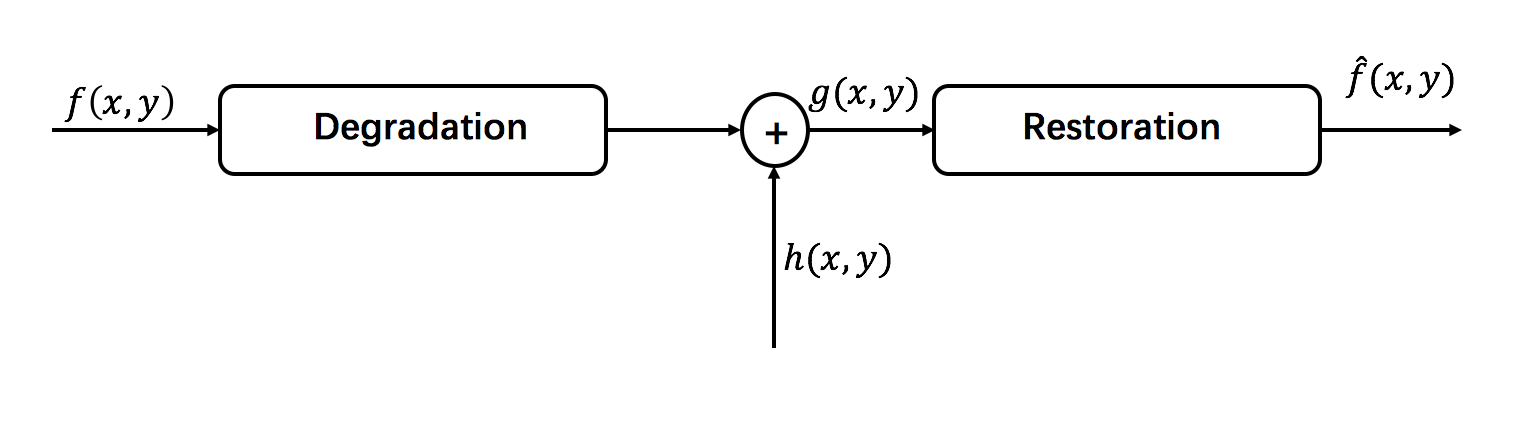$\begin{equation} \text{spatial domain: }g(x,y) = h(x,y)\ast f(x,y)+n(x,y)\\ \text{Frequency domain: }G(\mu,\upsilon) = H(\mu,\upsilon)F(\mu,\upsilon)+N(\mu,\upsilon) \end{equation}$
• $f(x,y)$: original image.
• $g(x,y)$: observed image corrupted by degradation.
• $h(x,y)$: degradation filter, point spread function or impulse response of the imaging system. Model degradation as a convolution with linear, shift invariant filter.
• $\hat{f}(x,y)$: estimated image, compute from $g(x,y)$.
• $n(x,y)$: addictive noise.

The most challenging issue of image restoration is loss of information and noise. For image blurring, blurring acts as a low pass filter and attenuates higher spatial frequencies.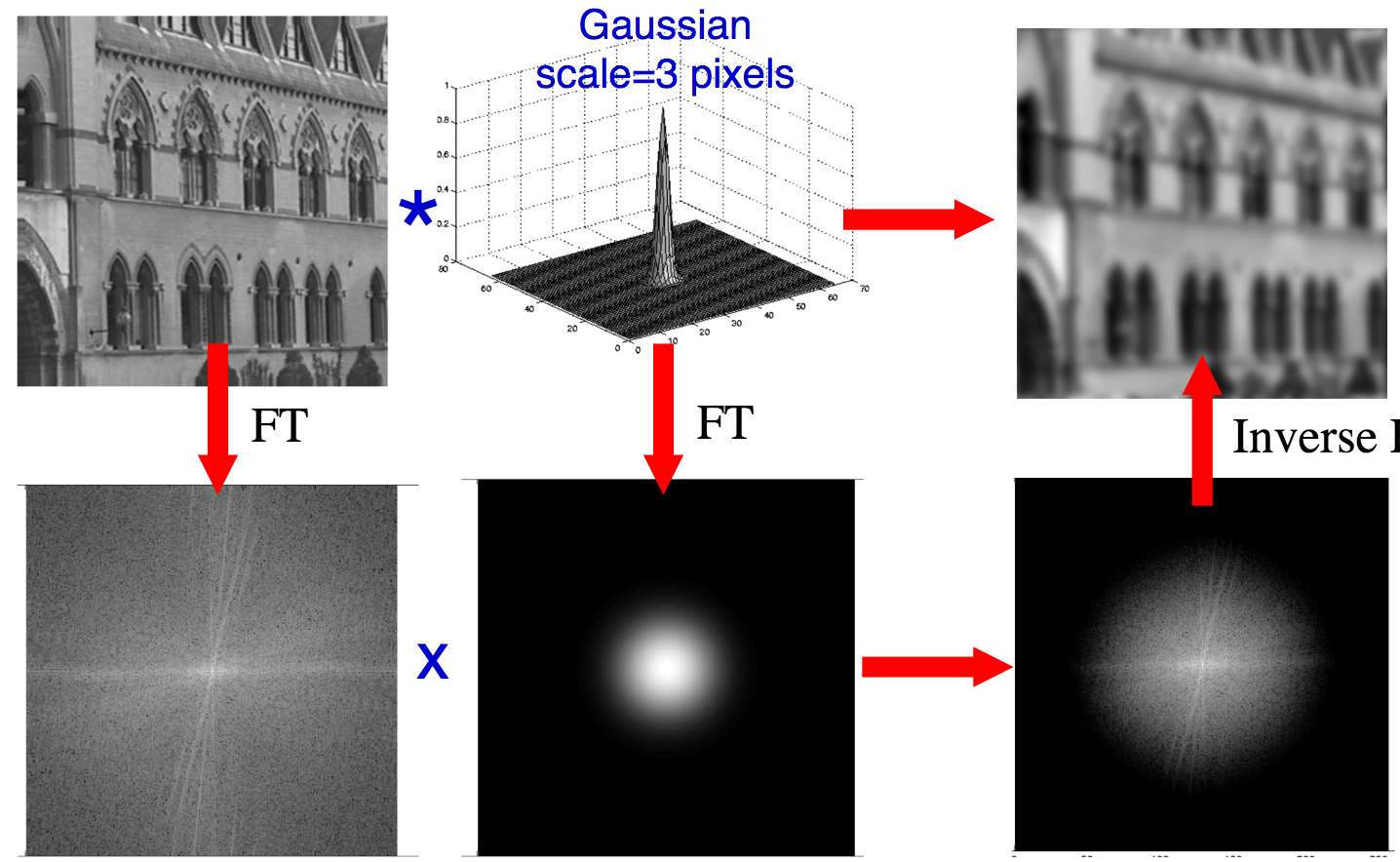## 2. The Inverse Filtter

From the perspective of frequency domain, image degradation can be modeled as: $G(\mu,\upsilon) = H(\mu,\upsilon)F(\mu,\upsilon)+N(\mu,\upsilon)$. To simplified the formulation, the noise term is ignored, and then the estimated function is obtained from inverse process:

$\hat{F}(x,y) = \frac{G(\mu,\upsilon)}{H(\mu,\upsilon)}$

The work flow of inverse filter: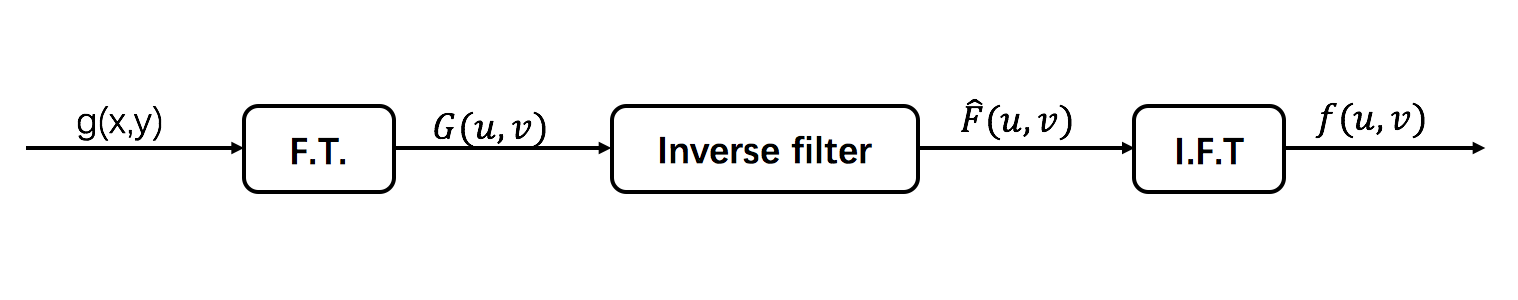The problem of noise amplification:

$\begin{equation} G(\mu,\upsilon) = H(\mu,\upsilon)F(\mu,\upsilon)+N(\mu,\upsilon)\\ \hat{F}(\mu,\upsilon)=\frac{G(\mu,\upsilon)}{H(\mu,\upsilon)} = F(\mu,\upsilon)+\frac{N(\mu,\upsilon)}{H(\mu,\upsilon)} \end{equation}$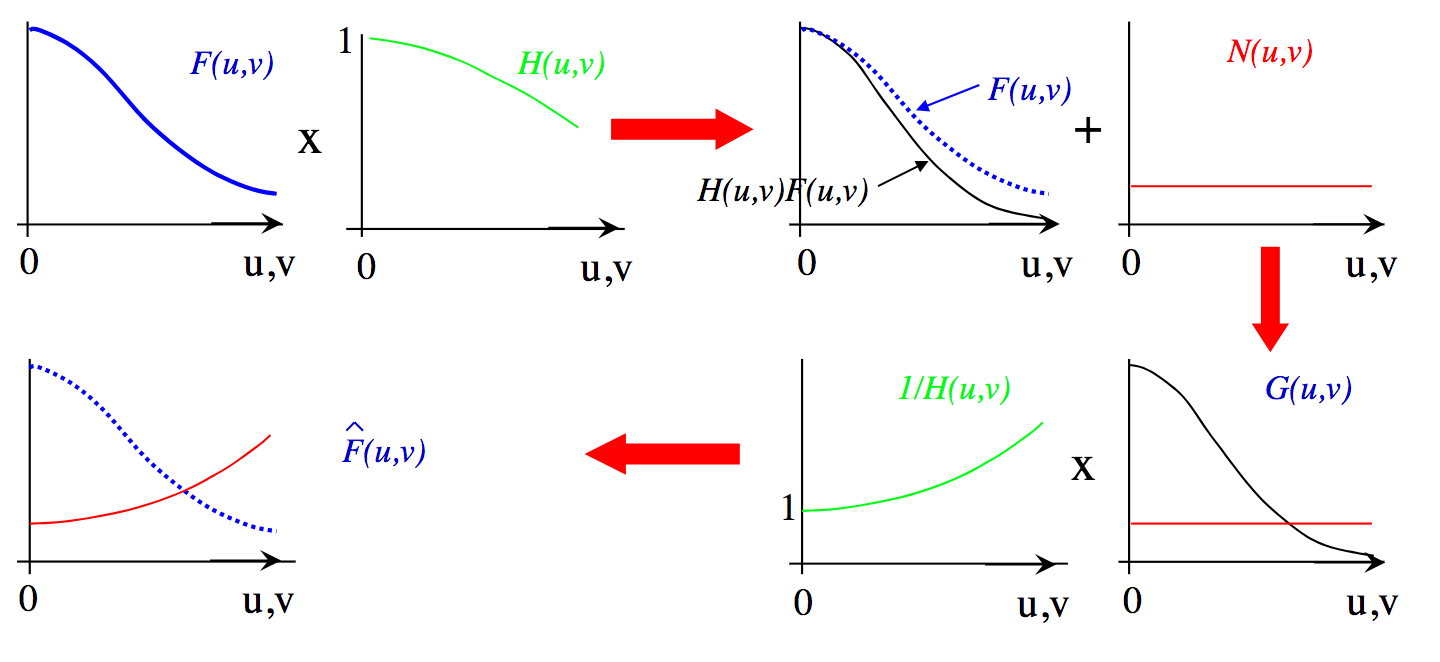## 3. The Wiener Filter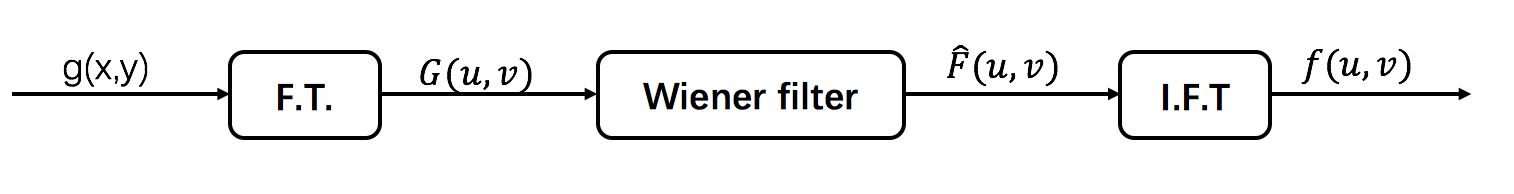A Wiener filter minimizes the least square error $\int_{-\infty}^{+\infty}\int_{-\infty}^{+\infty}(f(x,y)-\hat{f}(x,y))^{2}dxdy$, restoration with a wiener filter $W(\mu,\upsilon)$, detail proven is in appedix:

\begin{align} G(\mu,\upsilon) &= H(\mu,\upsilon)F(\mu,\upsilon)+N(\mu,\upsilon) \\ \hat{F}(\mu,\upsilon) &=W(\mu,\upsilon)G(\mu,\upsilon) \\ \label{eq:equation1} \end{align}

where $W(\mu,\upsilon)=\frac{H^{*}(\mu,\upsilon)}{|H(\mu,\upsilon)|^{2}+K(\mu,\upsilon)}$.

• $K(\mu,\upsilon)=\frac{S_{\eta}(\mu,\upsilon)}{S_{f}(\mu,\upsilon)}$, signal-noise-ratio.
• $S_{f}(\mu,\upsilon)=|F(\mu,\upsilon)|^{2}$ power spectral density of $f(x,y)$.
• $S_{\eta}(\mu,\upsilon)=|N(\mu,\upsilon)|^{2}$ power spectral density of $\eta(x,y)$.

Analysis of frequency behaviour: From the equation(4), we can conclude that:

• If $K=0$, then $W(\mu,\upsilon)=\frac{1}{H(\mu,\upsilon)}$, i.e. an inverse filter.
• If $K»|H(\mu,\upsilon)|$ for large $\mu,\upsilon$, then high frequency are attenuated.
• $|F(\mu,\upsilon)|$ and $N(\mu,\upsilon)$ are often known approximately and $K$ is set to a constant scalar which is determined empirically.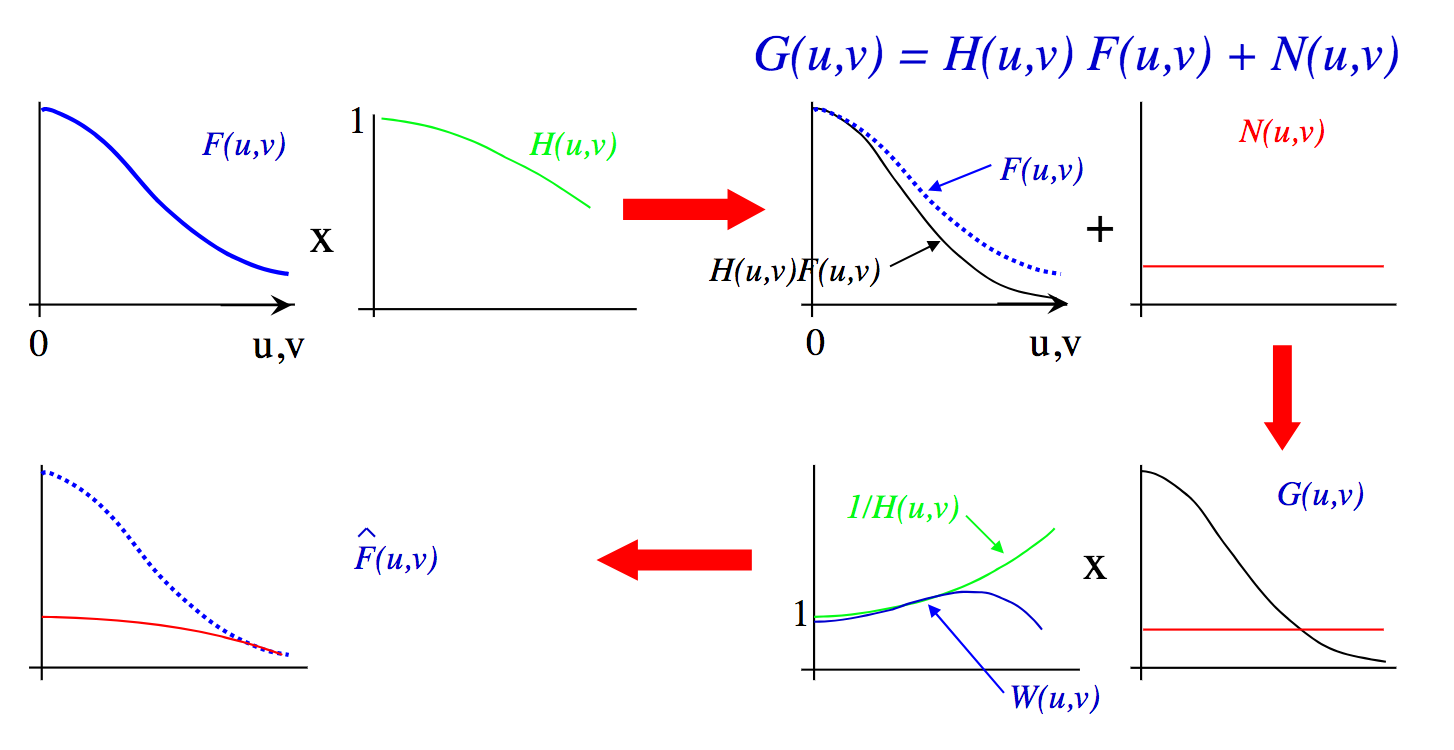## 4. MAP Formulation

Given an observed image $\hat{g}$ with $n$ pixels and unkonwn original image $f$, the degradation process can be formulated in the form of vector:

$\begin{equation} \hat{g} = Af + n \end{equation}$

where $\hat{g}$ and $f$ are image vector with n dimension, and $A$ is an $n\times n$ matrix.

To estimate $f(x,y)$ by optimizing a cost function:

$\begin{equation} \hat{f}=\text{arg }\min_{f}\Vert g-Af \Vert^{2} +\lambda p(f) \end{equation}$

where $\Vert g- Af \Vert^{2}$ is likelihood function and $\lambda p(f)$ is a prior regularization term.

Continue….(To figure out how super-resolution work.)

## Appendix

Wiener filter essentially aims to minimize the least square error, and the proven is shown in detail:

$\begin{equation} \varepsilon = \int_{-\infty}^{+\infty}\int_{-\infty}^{+\infty}(f(x,y)-\hat{f}(x,y))^{2} dxdy \end{equation}$

According to Paeawval’s theorem:

\begin{align} \varepsilon &= \int_{-\infty}^{+\infty}\int_{-\infty}^{+\infty}(f(x,y)-\hat{f}(x,y))^{2} dxdy \\ \varepsilon &= \int_{-\infty}^{+\infty}\int_{-\infty}^{+\infty}(F(\mu,\upsilon)-\hat{F}(\mu,\upsilon))^{2} d\mu d\upsilon \\ \end{align}

where

\begin{align} \hat{F}&=WG=WHF+WN \\ F-\hat{F} &= (1-WH)F-WH \\ \end{align}

Therefore

\begin{align} \varepsilon &= \int_{-\infty}^{+\infty}\int_{-\infty}^{+\infty}|(1-WH)F-WN|^{2} d\mu d\upsilon \\ &= \int_{-\infty}^{+\infty}\int_{-\infty}^{+\infty}|(1-WH)F|^{2}-|WN|^{2} d\mu d\upsilon \\ \end{align}

since $f(x,y)$ and $\eta$ are uncorrelated. Note:integrand is sum of two squares.

take a derivative to minimize the least square error:

$\begin{equation} \frac{\partial}{\partial z}2(-(1-W^{\ast}H^{\ast})H|F|^{2}+W^{\ast}|N|^{2})=0 \end{equation}$ \begin{align} W^{\ast} &=\frac{H|F|^{2}}{|H|^{2}|F|^{2}+|N|^{2}} \\ W^{\ast} &=\frac{H}{|H|^{2}+\frac{|N|^{2}}{|F|^{2}}} \end{align}